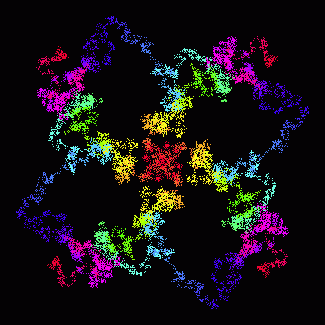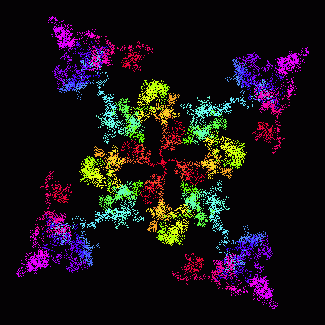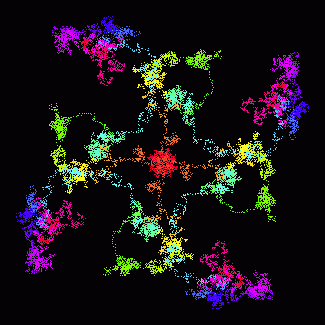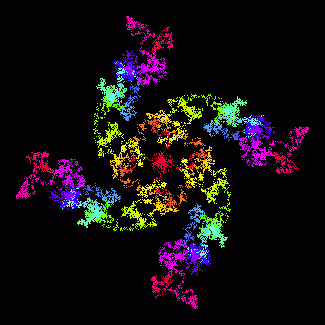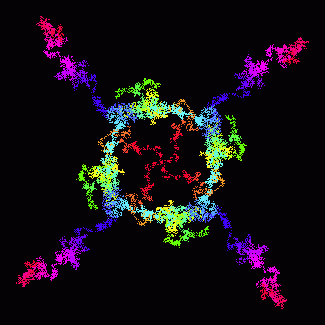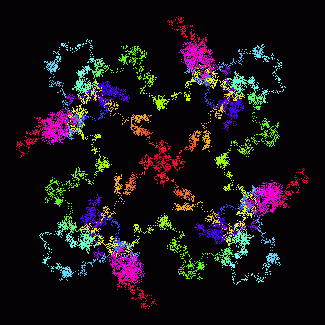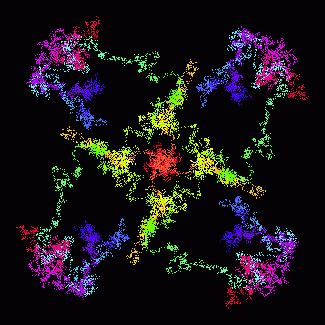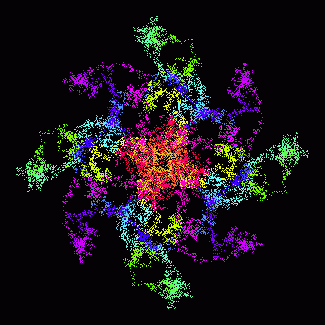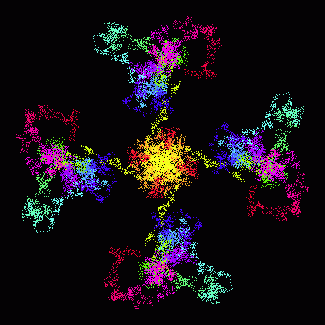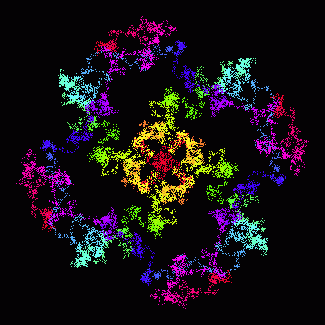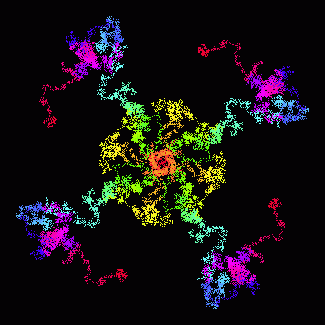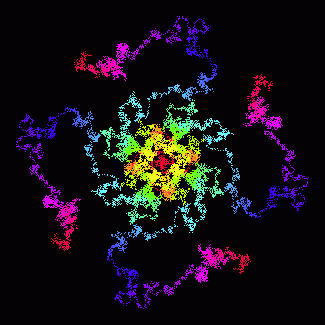The Fractal I Ching

This is experimental.  It generates hexagrams using a simple fractal algorithm, a modification of the Martin attractor by Barry Martin.  (For a more accessible and functional method using the same fractal, see Another Fractal I Ching.)

 Enter strings of digits into the fields below: x = y = a = b =  best if greater than 3 c = i =   may run slow Generate Use the system time and a random number Fractal size:  small  large  none
The raw numbers:  x, y, distance from the origin

Press Generate and the script will use the numbers in the form fields to generate the hexagram lines, which are displayed in the field to the right.  Below it is a graphic representation of the “fractal landscape” that the line numbers were based on, a small version of the actual fractal, and the hexagram reference(s).  Make any change to the parameters in the form to get different results.  The x value will be incremented if you simply press Generate over and over.  Check the box and the form will automatically use the system time and a random number as x and y parameters.

The algorithm is an iteration of the following:

x1 = y0 - sin((b * x0) - c)
y1 = a - x0

The equations work best when the initial x and y are small nonzero numbers, a is small, and b is greater than 3; c can be any number.  The strings of digits in the x, y, and a fields are turned into mantissas (digits after the decimal place; 1380502597083 becomes 0.1380502597083, for example).  The i value is the number of iterations performed prior to reading the data; it should be a positive integer.  Large i values (over 1000000 or so on my system) may run slow.  If you are using a fast browser, you can try the large fractal size, at the expense of speed.  (Besides using up more resources on the page, the large fractal is actually based on up to 10000 iterations, the small on up to 2000.)  The algorithm, as fractals are, is very sensitive to the slightest change in the equation parameters.  And the results are chaotic, not random.  Larger examples of fractals generated by the algorithm are shown at the upper right, and in the image gallery below.  Download Mandala Explorer if you want to play with it some more.  Why is this a fractal?

The points generated by the algorithm tend to loop around the origin quadrant by quadrant.  What the hexagram generator actually does is start with the x0 and y0 values entered into the form, then iterates the algorithm i times.  This lead-in determines the point in the sequence at which the data are read, and stabilizes the equations a bit.  It then records the distance from the origin (the square root of x2 + y2) of every 4th point, 14 times (which tends to be 14 consecutive points in the same quadrant).  The first and last points are used as a baseline, which is then moved to the center of the range of distances. The script then looks at the values of each distance to see if each point is above or below the baseline.  What we are left with is a representation of the “jaggedness” of the series of values.  Beginning with the second point, each of the six consecutive pairs of values between the ends is used to determine a hexagram line.  The first member of each pair determines the line type, positive = yang and negative = yin.  If the sign of the second member of the pair is opposite the first, the line is a moving line (this tends to result in about 1/4 of the lines moving).

= 7   = 9   = 8   = 6

Landscape patterns

Generating hexagrams based on a fractal landscape opens up a whole new way of viewing the line patterns.  Some tend to be wave-like:

777988
34  Great Strength
11  Peace
798867
41  Decrease
42  Increase

Every now and then there is a simple straight line:

888777
12  Obstruction

Many others are very chaotic, with multiple moving lines:

697667
18  Corruption
13  Gathering

Like I said, this is experimental.  The results have about the correct distribution of lines (1.1 : 2.9 : 2.9 : 1.1), but they tend to cluster a little.  For example, the number of identical quadruplets should be about 3%, but tends to be more like 4%.  This is perhaps what is to be expected from a fractal landscape distribution, rather than a random one.  More significantly, certain hexagrams appear much more often than others; the broadly-patterned hexagrams 11, 12, 19, 20, 33, and 34 account for about 23% of the total, while 27, 28, 31, 32, 41, and 42 account for another 20%.  The finely-patterned hexagrams 29, 30, 37, 38, 39, 40, 63, and 64 account for only about 5%.

A more accurate way of using fractal points to generate lines is to measure them in threes and treat them like coin tosses:  for example, heads if the point moves away from the origin, tails if it moves back.  But this is not as graphic as the method above.  For a even better method, see Another Fractal I Ching.

Should work in any modern browser.  Updated 9/28/2019.

Image gallery